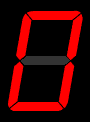# Drive a 7-Segment Display With Your FPGA

## Convert from Binary in VHDL and Verilog

A Seven-Segment Display is an indicator commonly used by FPGA designers to show information to the user. Code to convert from binary to seven-segment display compatible can be done easily in VHDL and Verilog. There are many applications that can require the use of one or more seven-segment displays such as:

• Alarm Clock
• Stop Watch
• Button Count Indicator
• Voltage Measurements (from Analog to Digital Converter)
• Many more!The GIF on the right is taken from the Wikipedia article about 7-segment displays. It shows what the code below will display to the user. The code takes in a 4-bit binary input. This allows for the decimal numbers 0-9 and the Hex Characters A-F to be displayed to the user.

Both the VHDL and Verilog code work the same way. They simply use a Look-Up Table to do the decoding from the hexadecimal input to the 7-segment output. The individual segments are listed in the table below.

7-Segment Locations
Name Location
A Top Middle
B Top Right
C Bottom Right
D Bottom Middle
E Bottom Left
F Top Left
G Middle
DP Decimal PointNote that the code below will only drive one seven-segment display and therefore can only display decimal numbers 0-9. Any application requiring a number higher than nine will need to first convert the number to Binary Coded Decimal (BCD). The linked code shows how this is done. If this is your first time using a seven-segment display you should just try counting 0-9 first, then work your way up to a 2-digit display.

### VHDL Implementation:

```-------------------------------------------------------------------------------
-- File downloaded from http://www.nandland.com
-------------------------------------------------------------------------------
-- This file converts an input binary number into an output which can get sent
-- to a 7-Segment LED.  7-Segment LEDs have the ability to display all decimal
-- numbers 0-9 as well as hex digits A, B, C, D, E and F.  The input to this
-- module is a 4-bit binary number.  This module will properly drive the
-- individual segments of a 7-Segment LED in order to display the digit.
-- Hex encoding table can be viewed at:
-- http://en.wikipedia.org/wiki/Seven-segment_display
-------------------------------------------------------------------------------
library ieee;
use ieee.std_logic_1164.all;

entity Binary_To_7Segment is
port (
i_Clk        : in  std_logic;
i_Binary_Num : in  std_logic_vector(3 downto 0);
o_Segment_A  : out std_logic;
o_Segment_B  : out std_logic;
o_Segment_C  : out std_logic;
o_Segment_D  : out std_logic;
o_Segment_E  : out std_logic;
o_Segment_F  : out std_logic;
o_Segment_G  : out std_logic
);
end entity Binary_To_7Segment;

architecture RTL of Binary_To_7Segment is

signal r_Hex_Encoding : std_logic_vector(7 downto 0) := (others => '0');

begin

-- Purpose: Creates a case statement for all possible input binary numbers.
-- Drives r_Hex_Encoding appropriately for each input combination.
process (i_Clk) is
begin
if rising_edge(i_Clk) then
case i_Binary_Num is
when "0000" =>
r_Hex_Encoding <= X"7E";
when "0001" =>
r_Hex_Encoding <= X"30";
when "0010" =>
r_Hex_Encoding <= X"6D";
when "0011" =>
r_Hex_Encoding <= X"79";
when "0100" =>
r_Hex_Encoding <= X"33";
when "0101" =>
r_Hex_Encoding <= X"5B";
when "0110" =>
r_Hex_Encoding <= X"5F";
when "0111" =>
r_Hex_Encoding <= X"70";
when "1000" =>
r_Hex_Encoding <= X"7F";
when "1001" =>
r_Hex_Encoding <= X"7B";
when "1010" =>
r_Hex_Encoding <= X"77";
when "1011" =>
r_Hex_Encoding <= X"1F";
when "1100" =>
r_Hex_Encoding <= X"4E";
when "1101" =>
r_Hex_Encoding <= X"3D";
when "1110" =>
r_Hex_Encoding <= X"4F";
when "1111" =>
r_Hex_Encoding <= X"47";
end case;
end if;
end process;

-- r_Hex_Encoding(7) is unused
o_Segment_A <= r_Hex_Encoding(6);
o_Segment_B <= r_Hex_Encoding(5);
o_Segment_C <= r_Hex_Encoding(4);
o_Segment_D <= r_Hex_Encoding(3);
o_Segment_E <= r_Hex_Encoding(2);
o_Segment_F <= r_Hex_Encoding(1);
o_Segment_G <= r_Hex_Encoding(0);

end architecture RTL;
```

### Verilog Implementation:

```///////////////////////////////////////////////////////////////////////////////
// File downloaded from http://www.nandland.com
///////////////////////////////////////////////////////////////////////////////
// This file converts an input binary number into an output which can get sent
// to a 7-Segment LED.  7-Segment LEDs have the ability to display all decimal
// numbers 0-9 as well as hex digits A, B, C, D, E and F.  The input to this
// module is a 4-bit binary number.  This module will properly drive the
// individual segments of a 7-Segment LED in order to display the digit.
// Hex encoding table can be viewed at:
// http://en.wikipedia.org/wiki/Seven-segment_display
///////////////////////////////////////////////////////////////////////////////

module Binary_To_7Segment
(
input       i_Clk,
input [3:0] i_Binary_Num,
output      o_Segment_A,
output      o_Segment_B,
output      o_Segment_C,
output      o_Segment_D,
output      o_Segment_E,
output      o_Segment_F,
output      o_Segment_G
);

reg [6:0]    r_Hex_Encoding = 7'h00;

// Purpose: Creates a case statement for all possible input binary numbers.
// Drives r_Hex_Encoding appropriately for each input combination.
always @(posedge i_Clk)
begin
case (i_Binary_Num)
4'b0000 : r_Hex_Encoding <= 7'h7E;
4'b0001 : r_Hex_Encoding <= 7'h30;
4'b0010 : r_Hex_Encoding <= 7'h6D;
4'b0011 : r_Hex_Encoding <= 7'h79;
4'b0100 : r_Hex_Encoding <= 7'h33;
4'b0101 : r_Hex_Encoding <= 7'h5B;
4'b0110 : r_Hex_Encoding <= 7'h5F;
4'b0111 : r_Hex_Encoding <= 7'h70;
4'b1000 : r_Hex_Encoding <= 7'h7F;
4'b1001 : r_Hex_Encoding <= 7'h7B;
4'b1010 : r_Hex_Encoding <= 7'h77;
4'b1011 : r_Hex_Encoding <= 7'h1F;
4'b1100 : r_Hex_Encoding <= 7'h4E;
4'b1101 : r_Hex_Encoding <= 7'h3D;
4'b1110 : r_Hex_Encoding <= 7'h4F;
4'b1111 : r_Hex_Encoding <= 7'h47;
endcase
end // always @ (posedge i_Clk)

// r_Hex_Encoding is unused
assign o_Segment_A = r_Hex_Encoding;
assign o_Segment_B = r_Hex_Encoding;
assign o_Segment_C = r_Hex_Encoding;
assign o_Segment_D = r_Hex_Encoding;
assign o_Segment_E = r_Hex_Encoding;
assign o_Segment_F = r_Hex_Encoding;
assign o_Segment_G = r_Hex_Encoding;

endmodule // Binary_To_7Segment
```

Help Me Make Great Content!     Support me on Patreon!     Buy a Go Board!